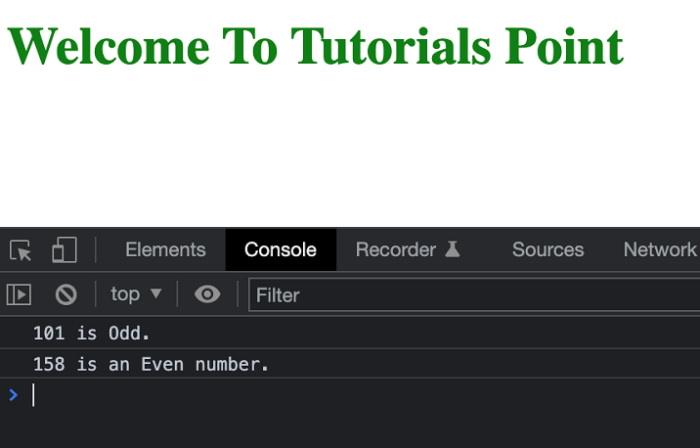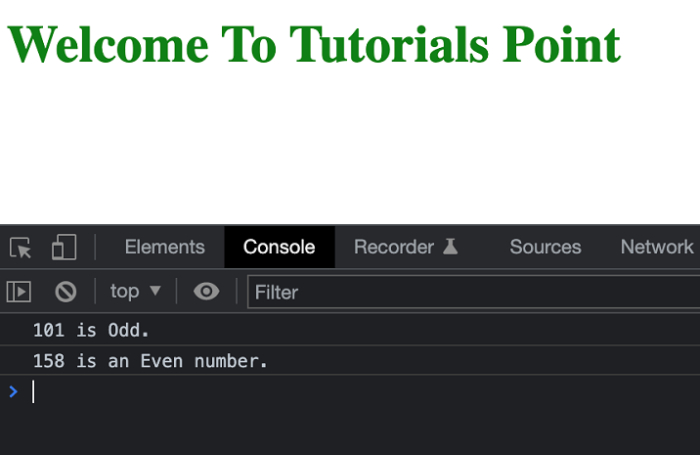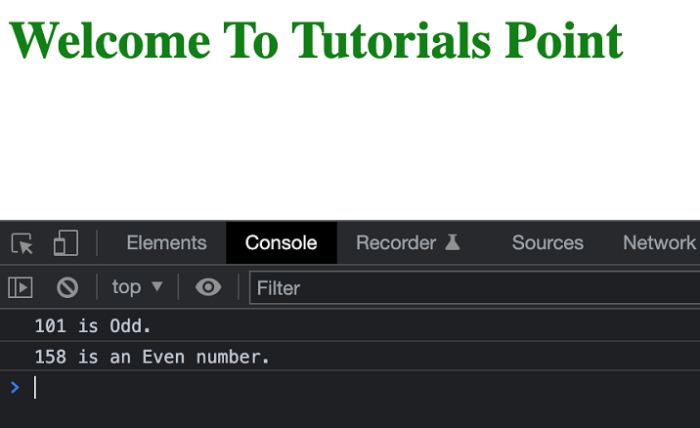# JavaScript: How to check if a number is even without using the modulo operator?

Often we need to check whether a number is odd or even and return the same to the user. In this article also we will be checking whether a number is even or not but without using the modulo operator. It’s pretty easy to check whether a number is even by using the modulo operator. But we can also check a number with other possible methods.

Below we are going to see the other methods for checking a number and its properties.

## Approach #1: Using the for() loop

In this method, we are going to use the for() loop to check whether a number is even or not. The idea is to take a Boolean flag variable as true and check it up to n times. If the flag gets its value back it means the value was even else not.

## Example 1

In the below example, we are going to check whether a number is even or not by using the for() loop approach.

# index.html

<!DOCTYPE html>
<html>
<title>Checking If a Number is Even</title>
<body>
<h1 style="color: green">
Welcome To Tutorials Point
</h1>
<script>
// Returns true if n is even,
function isEven(n) {
let isEven = true;
for(let i = 1; i <= n; i++)
isEven = !isEven;
if (isEven)
console.log(n + " is an Even number.");
else
console.log(n + " is Odd.");
}
// Driver Code
isEven(101);
isEven(158);
</script>
</body>
</html>

## Output

The above program will produce the result in the console similar to the below screenshot −## Approach #2: Using multiplication and division

In this method, we are going to divide a number by 2 and then multiply the result by 2. If the result is the same as that of the original number, it is an even number.

## Example 2

In the below example, we are going to check whether a number is even or not by using multiplication and division.

# index.html

<!DOCTYPE html>
<html>
<title>Checking If a Number is Even</title>
<body>
<h1 style="color: green;">
Welcome To Tutorials Point
</h1>
<script>
// Returns true if n is even,
function isEven(n) {
// Return true if n/2 does not result
// in a float value.
if(parseInt(n / 2, 10) * 2 == n) {
console.log(n + " is an Even number.");
} else {
console.log(n + " is Odd.");
}
}
// Driver Code
isEven(101);
isEven(158);
</script>
</body>
</html>

## Output

The above program will produce the result in the console similar to the below screenshot −## Approach #3: Using the bitwise operator

In this method, we are going to use the bitwise AND operator to check the number.

## Example #3

# index.html

<!DOCTYPE html>
<html>
<title>Checking If a Number is Even</title>
<body>
<h1 style="color: green;">
Welcome To Tutorials Point
</h1>
<script>
// Returns true if n is even,
function isEven(n) {
// n&1 is 1, then odd, else even
if (!(n & 1)) {
console.log(n + " is an Even number.");
} else {
console.log(n + " is Odd.");
}
}
// Driver Code
isEven(101);
isEven(158);
</script>
</body>
</html>

## Output